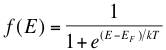# 4. Fermi Energy Levels

As discussed in “Band Gaps”, the valence and conduction bands represent groups of energy states of the electrons. However, according to something called the Pauli exclusion principle, a result of quantum mechanics, each allowed energy level can be occupied by no more than two electrons of opposite “spin”. This just means that, at low temperatures, all available states in the crystal up to a certain energy level will be occupied by two electrons1. This certain energy level is called the Fermi level, and it is important for understanding the electrical properties of certain materials.

The probability that electrons will occupy a certain energy level at a certain temperature is given by the Fermi-Dirac distribution function:where T is temperature, k is Boltzmann’s constant (1.38 x 10-23 Joules per Kelvin), EF is the Fermi energy level of a given material and E is the allowed energy state whose probability we are trying to find. This equation just means that as temperature increases, electrons are more likely to be found in the higher energy states. When the temperature is near absolute zero, we see that f(E) becomes 1, giving that nearly all the electrons are below the Fermi level (see below). At higher temperatures, energy levels in the conduction band have a higher probability of occupation and levels in the valence band are more likely to be empty. There is also an equation which allows us to solve for the Fermi level of a material using our knowledge of the band gap of that material, however we will not discuss that equation in detail here.Above we see that the distribution "smears" as the temperature rises. A huge difference between a conductor and semiconductor is that increasing temperature usually hinders current flow in conductors, while improving conductivity in semiconductors. This is due to the band gap and Fermi level of semiconductors.